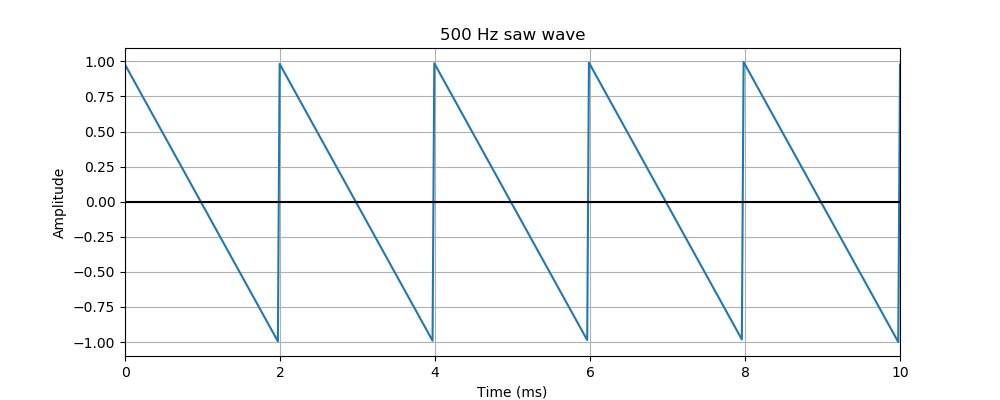# Pysound simple saw waves

Martin McBride
2021-10-03

In this example, we will create a simple saw (or triangle) wave.

This process is quite similar to the way we create a sine wave, it is worth reading that first.. The way we create a graph of the function, and the way we vary the amplitude and frequency are identical, so we won't repeat them here.

However, saw waves have an extra parameter, the ratio, that determines the rise/fall time of the wave. This creates waves with different shapes, and and similar but different sounds.

## The code

Here is the code:

from pysound.buffer import BufferParams
from pysound.oscillators import sine_wave
from pysound import soundfile
from pysound.graphs import Plotter

params = BufferParams()
out = saw_wave(params, frequency=500)
soundfile.save(params, '/tmp/triangle-500.wav', out)


This works in a similar way to the sine wave example, but it uses the saw_wave function instead:

out = saw_wave(params, frequency=500)


It is stored as triangle-500.wav in the current folder.

Here is what the sound looks like:The terms triangle and saw are often used interchangeably. Technically a saw wave is a special case of a triangle wave, that has either a rise time of 0 or a fall time of 0. We will see an example below. The wave above is more accurately described as a triangle wave rather than a saw wave.

This sound is a harsher and more electronic sounding than a sine wave, but less harsh than a square wave.

## Changing the ratio of the triangle wave

A triangle wave rises linearly to its maximum value (+1.0 in this case) then falls linearly to its minimum value (-1.0 in this case) . By default, it spends half its time rising, and half its time falling.

We can change this using the ratio parameter. The ratio specifies the on time as a proportion of the total wave time. A square wave, above, has a ratio of 0.5 (it is on for 50% of the time, therefore off for 50% of the time). The ratio parameter defaults to 0.5, so if you don;t specify it you will get a square wave.

We can change the ratio like this:

out = saw_wave(params, frequency=500, ratio=0.3)
soundfile.save(params, 'triangle-unequal-500.wav', out)


In this case, the ratio is 0.3 (it rises for 30% of the time, and falls for 70% of the time).

A wave with unequal rise/fall times is still called a triangle wave. Here is what the 0.3 case looks like:## Saw wave

As we mentioned above, a saw wave is a special case of a triangle wave. We can created it by setting the ratio to 0, so the rise time is 0. Here is the code:

out = saw_wave(params, frequency=500, ratio=0)
soundfile.save(params, '/tmp/saw-500.wav', out)


Here is what it looks like:The wave starts at +1.0, falls linearly to -1.0, the immediately jumps to +1.0 and starts again. This discontinuous jump in value is similar to a square wave.

If we were to set the ratio to 1 rather than 0, the wave would spend all of its time rising linearly form -1.0 to +1.0, then jump back to -1.0. It would be like the wave above, turned upside down, and it would sound identical.